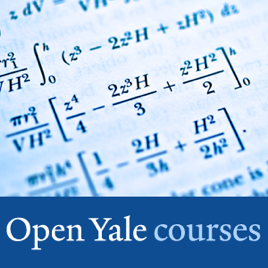24 episodes

(PHYS 200) This course provides a thorough introduction to the principles and methods of physics for students who have good preparation in physics and mathematics. Emphasis is placed on problem solving and quantitative reasoning. This course covers Newtonian mechanics, special relativity, gravitation, thermodynamics, and waves.

This class was recorded in Fall 2006.# Physics - Video Yale University

• Science

(PHYS 200) This course provides a thorough introduction to the principles and methods of physics for students who have good preparation in physics and mathematics. Emphasis is placed on problem solving and quantitative reasoning. This course covers Newtonian mechanics, special relativity, gravitation, thermodynamics, and waves.

This class was recorded in Fall 2006.

• video
01 - Course Introduction and Newtonian Mechanics

## 01 - Course Introduction and Newtonian Mechanics

Professor Shankar introduces the course and answers student questions about the material and the requirements. He gives an overview of Newtonian mechanics and explains its two components: kinematics and dynamics. He then reviews basic concepts in calculus through two key equations: x = x0 + v0t + ½ at2 and v2 = v02+ 2 a (x-x0), tracing the fate of a particle in one dimension along the x-axis.

• 1 hr 13 min
• video
02 - Vectors in Multiple Dimensions

## 02 - Vectors in Multiple Dimensions

In this lecture, Professor Shankar discusses motion in more than one dimension. Vectors are introduced and discussed in multiple dimensions. Vector magnitude and direction are also explained. Null vectors, minus vectors, unit and velocity vectors are discussed along with their properties. Finally, several specific problems are solved to demonstrate how vectors can be added, and problems of projectile motion are expounded.

• 1 hr 6 min
• video
03 - Newton's Laws of Motion

## 03 - Newton's Laws of Motion

This lecture introduces Newton's Laws of Motion. The First Law on inertia states that every object will remain in a state of rest or uniform motion in a straight line unless acted upon by an external force. The Second Law (F = ma) relates the cause (the force F) to the acceleration. Several different forces are discussed in the context of this law. The lecture ends with the Third Law which states that action and reaction are equal and opposite.

• 1 hr 8 min
• video
04 - Newton's Laws (cont'd) and Inclined Planes

## 04 - Newton's Laws (cont'd) and Inclined Planes

The lecture begins with the application of Newton's three laws, with the warning that they are not valid for objects that move at speeds comparable to the speed of light or objects that are incredibly small and of the atomic scale. Friction and static friction are discussed. The dreaded inclined plane is dealt with head on. Finally, Professor Shankar explains the motion of objects using Newton's laws in specific problems related to objects in circular motion, such as roller coasters and a planet orbiting the Sun.

• 1 hr 7 min
• video
05 - Work-Energy Theorem and Law of Conservation of Energy

## 05 - Work-Energy Theorem and Law of Conservation of Energy

The lecture begins with a review of the loop-the-loop problem. Professor Shankar then reviews basic terminology in relation to work, kinetic energy and potential energy. He then goes on to define the Work-Energy Theorem. Finally, the Law of Conservation of Energy is discussed and demonstrated with specific examples.

• 1 hr 10 min
• video
06 - Law of Conservation of Energy in Higher Dimensions

## 06 - Law of Conservation of Energy in Higher Dimensions

The discussion on the Law of Conservation of Energy continues but is applied in higher dimensions. The notion of a function with two variables is reviewed. Conservative forces are explained and students are taught how to recognize and manufacture them.

• 1 hr 11 min